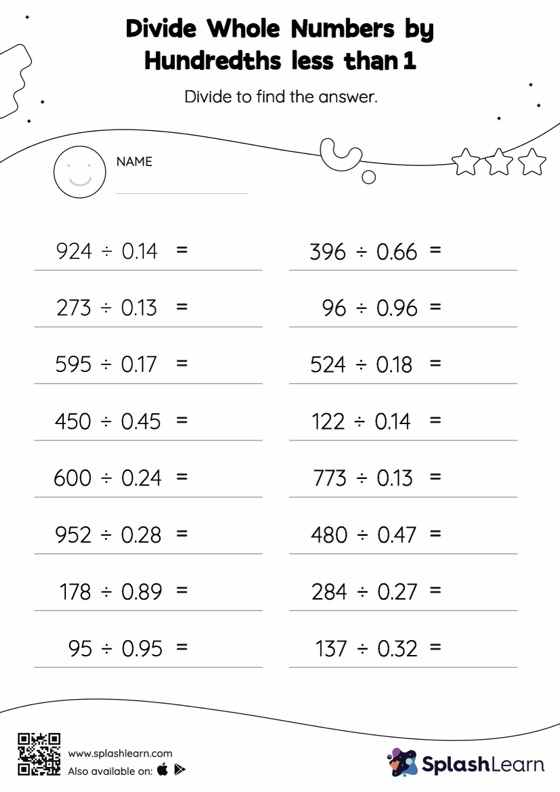# Divide Whole Numbers by Hundredths less than 1: Horizontal Division Worksheet

Home > Divide Whole Numbers by Hundredths less than 1: Horizontal DivisionDivide whole numbers by hundredths less than 1 worksheet helps students develop proficiency with division. Students divide a whole number by a decimal without taking the decimal point into account, and then, depending on the number of decimal digits in the divisor, they move each digit of the quotient to the left. Students practice this concept in great depth with divide whole numbers by hundredths less than 1 worksheet.